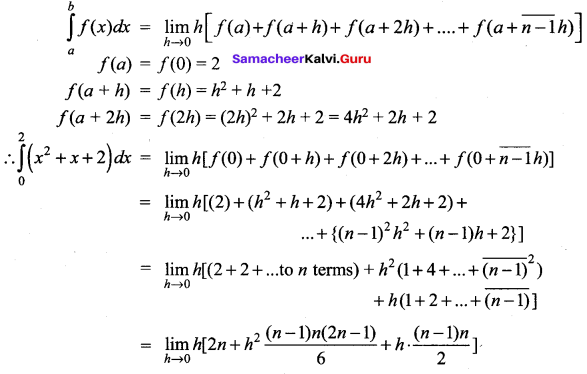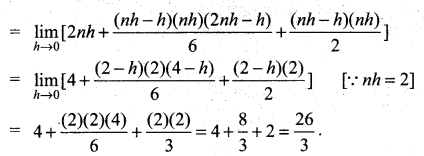# Samacheer Kalvi 12th Maths Solutions Chapter 9 Applications of Integration Ex 9.2

## Tamilnadu Samacheer Kalvi 12th Maths Solutions Chapter 9 Applications of Integration Ex 9.2

Question 1.
Evaluate the following integrals as the limits of sums: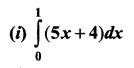Solution:
We use the formula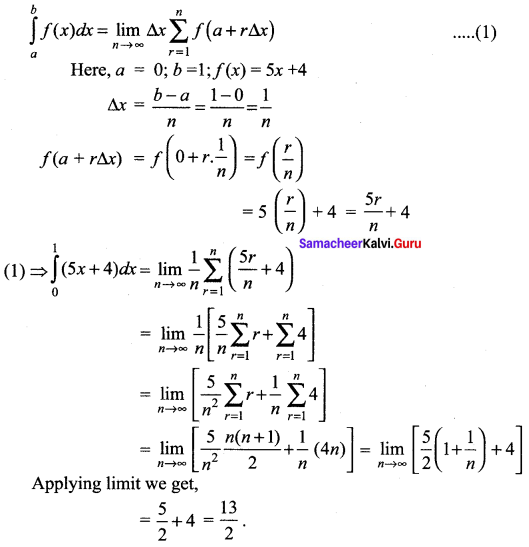(ii) $$\int_{1}^{2}\left(4 x^{2}-1\right) d x$$
Solution:
We use the formula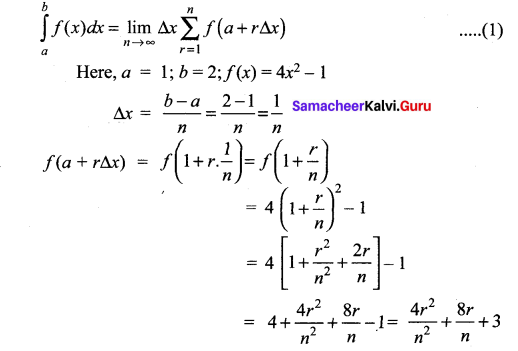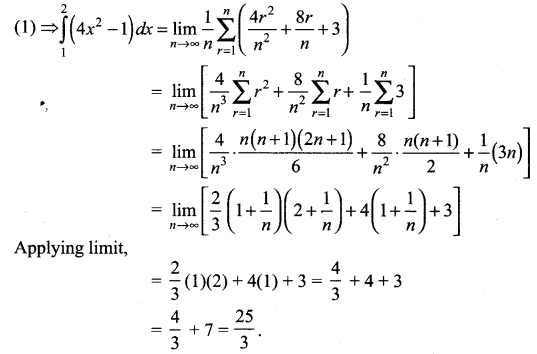### Samacheer Kalvi 12th Maths Solutions Chapter 9 Applications of Integration Ex 9.2 Additional Problems

Question 1.
Evaluate as the limit of sums: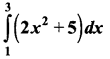Solution:
Let f(x) = 2x2 +5; a = 1; b = 3 and nh = 3 – 1 = 2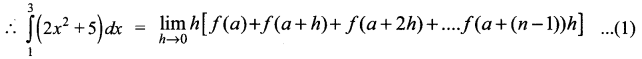Here, f(a) = f(1) = 2(1)2 + 5
f(a + h) = f(1 + h) = 2(1 + h)2 + 5
f(a + 2h) = f(1 + 2h) = 2 (1 + 2h)2 + 5
f[a+(n – 1)h = f[1 + (n – 1)h] = 2[1 + (n – 1)h]2 + 5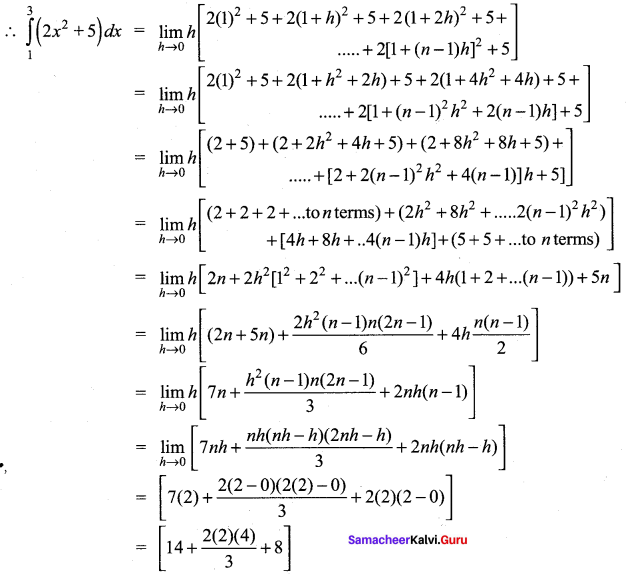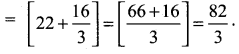Question 2.
Evaluates as the limit of sums: $$\int_{1}^{2}\left(x^{2}-1\right) d x$$
Solution:
Let f(x) = x2 – 1 for 1 ≤ x ≤ 2
We divide the interval [1, 2] into n equal sub-intervals each of length h.
We have a = 1, b = 2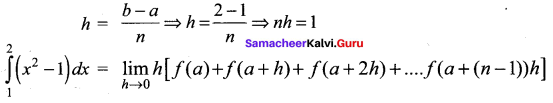Here, f(a) = f(1) = (1)2 – 1 = 0
f(a + h) = f(1 + h) = (1 + h)2 – 1 = 1 + h2 + 2h – 1 = h2 + 2h
f(a + 2h) = f(1 + 2h) = (1 + 2h)2 – 1 = 1 + 4h2 + 4h – 1 = 4h2 + 4h
f[a + (n -1)h] = f[1 + (n – 1)h] = [1 + (n – 1)h]2 – 1
= 1 + (n – 1)2 h2 + 2(n – 1 )h – 1 = (n – 1)2 h2 + 2 (n – 1 )h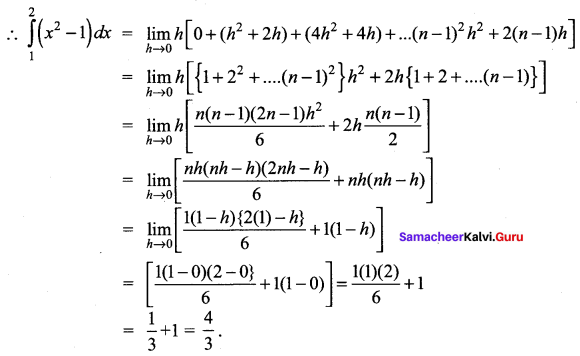Question 3.
Evalute: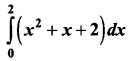Solution:
Let f(x) = x2 + x + 2, for 0 ≤ x ≤ 2 Here, a = 0,b = 2
We divide the closed interval [0, 2] into n subintervals each of length h.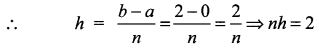By definition,